# Linear Graph

Linear Graph

In this mini-lesson, we will explore the world of linear graphs. Starting with the definition, we will understand the properties of linear graphs, their equations and learn how to plot or analyze them.

Before we do all of that, let's hear James' story.

James was offered a job where he would be paid $15 per hour. He has an average daily expenditure of$50 The graph will be similar to what is given below. Don't worry, we will explain it in detail in the coming section.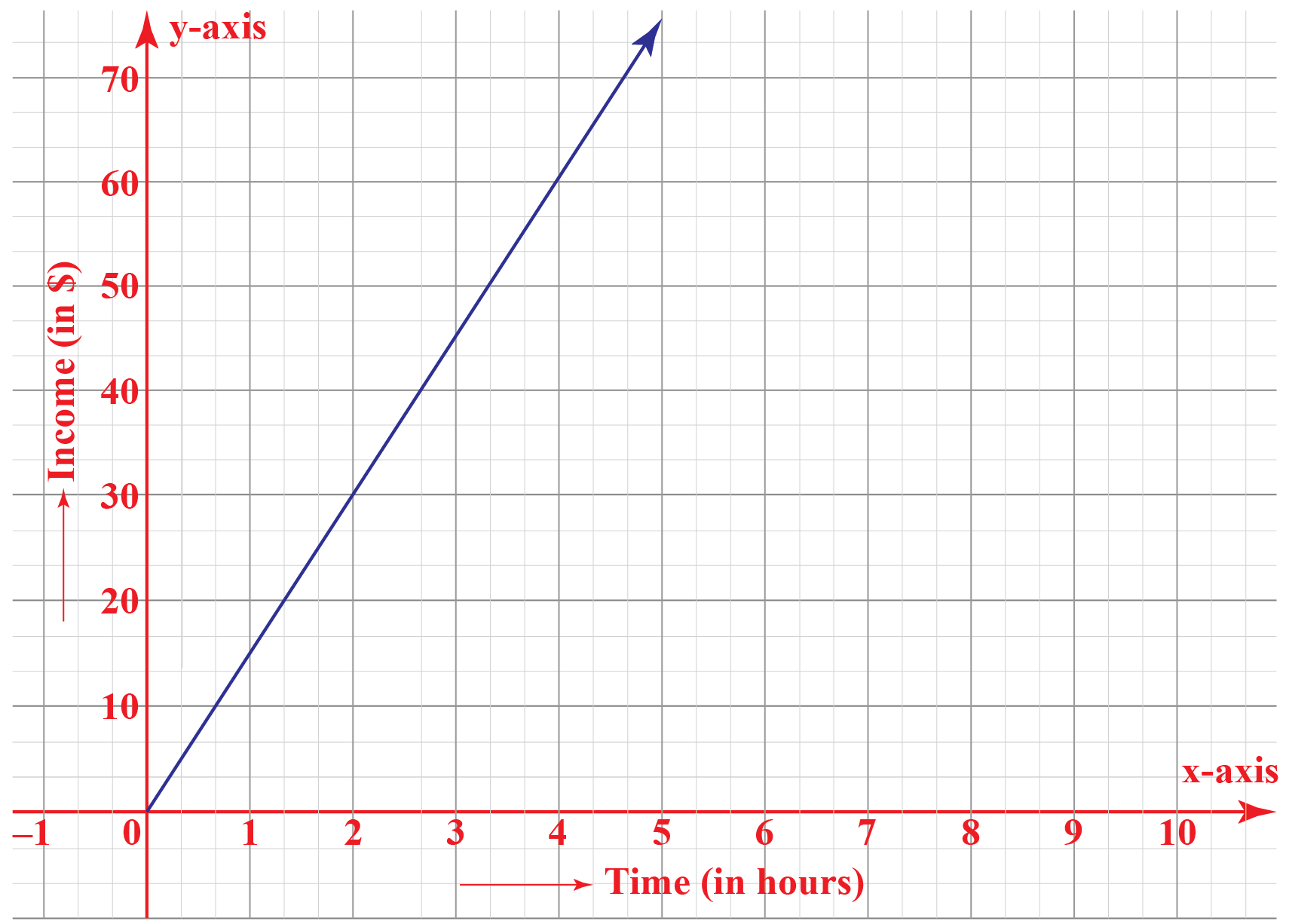He wants to know, how many minimum hours he should work in a day so that he has some savings.

Can you think of different ways of finding out this?

Let's study how a linear graph can answer this problem.

## Lesson Plan

 1 What Is Meant by Linear Graph? 2 Solved Examples on Linear Graph 3 Interactive Questions on Linear Graph

## What Is Meant by Linear Graph?

The word "linear" stands for a line.

"A linear graph is the graphical representation of a straight line."

It showcases a linear equation in two variables and shows the linear association between two quantities.

The case that we witnessed in the introduction section is one of the linear graph examples.

Let's have a look at the same graph, again. If we represent time by $$t$$ and income by $$I$$, we get a linear equation:

$$I=15t$$On analyzing the graph, we can find out the value on the vertical axis which is just more than his expenditure of $50 Now, draw a horizontal line through that value and drop a vertical line to intersect the horizontal axis. This value on the horizontal axis gives the minimum number of hours James should work to have some savings every day. After having knowledge of linear graph meaning, let's understand how it's different from a line graph. ### Linear Graph vs Line Graph Look at some of the graphs.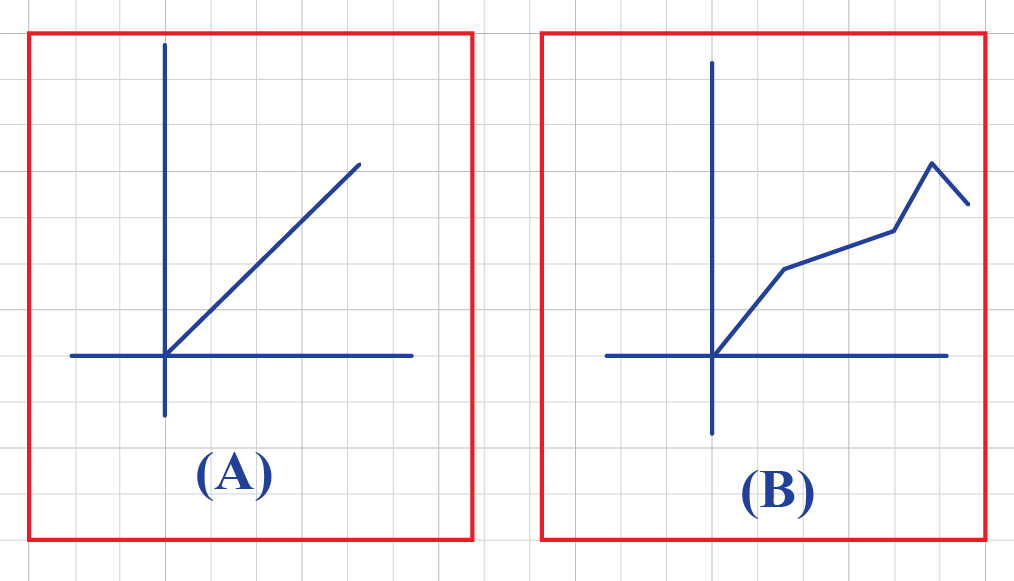In the figure given above, can you make out how B is different from A? Here, A is a linear graph whereas B represents a line graph. Though both of them are made up of line segments, there is a major difference between them. The difference lies in the figure obtained after joining the line segments. All the points in a line graph are collinear and hence lie on a line. But in the case of a line graph, they may or may not be collinear. ## What Is Linear Graph Equation? A linear graph is the graphical representation of a linear equation. A linear equation is an equation which can be written in the form:  $$Ax + By = C$$ for some real numbers A, B, and C where A and B, are not 0 For example, the linear equation $$2x+5y = 0$$ on a graph looks like this: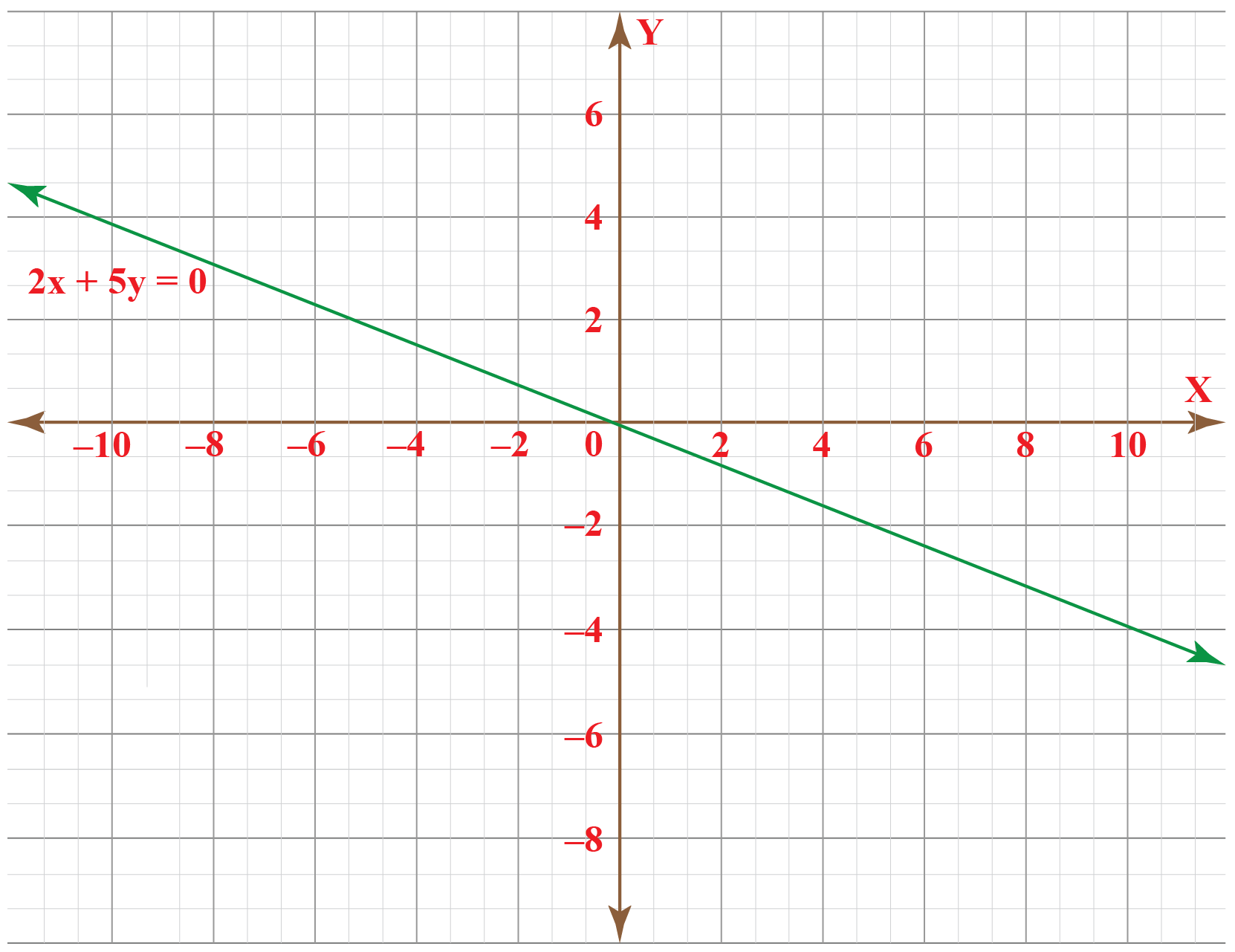Think Tank 1. Imagine that three friends bought a cake. The total worth of the cake is$30 1st friend contributed \$10 Can you represent this situation as a linear graph?
2. If the 2nd friend's contribution was one-third of contribution by 3rd friend, what was each of their contribution?

## How To Plot Linear Equation On a Graph?

Consider the following steps to plot a linear equation on a graph:

1. Identify the two quantities which are varying.

Let the 1st quantity be $$x$$ and the 2nd quantity be $$y.$$

2. Next, find out three ordered pairs (x,y) which satisfies the given equation.

3. Present these values in a tabular form.

4. Plot the points given in the table on a cartesian plane.

5. Join the points.

Example

$$2x + y = 6$$

To plot this on a graph we write it in the form $$y = mx + b$$.

So,

$$y = -2x + 6$$

To get the solution to the linear equation, we can plug in a numerical value to $$x$$ and obtain the corresponding value for $$y$$.

Three of the solutions are:

 x 0 1 -1 y 6 4 8

Now, plot these points on an XY-plane and join them.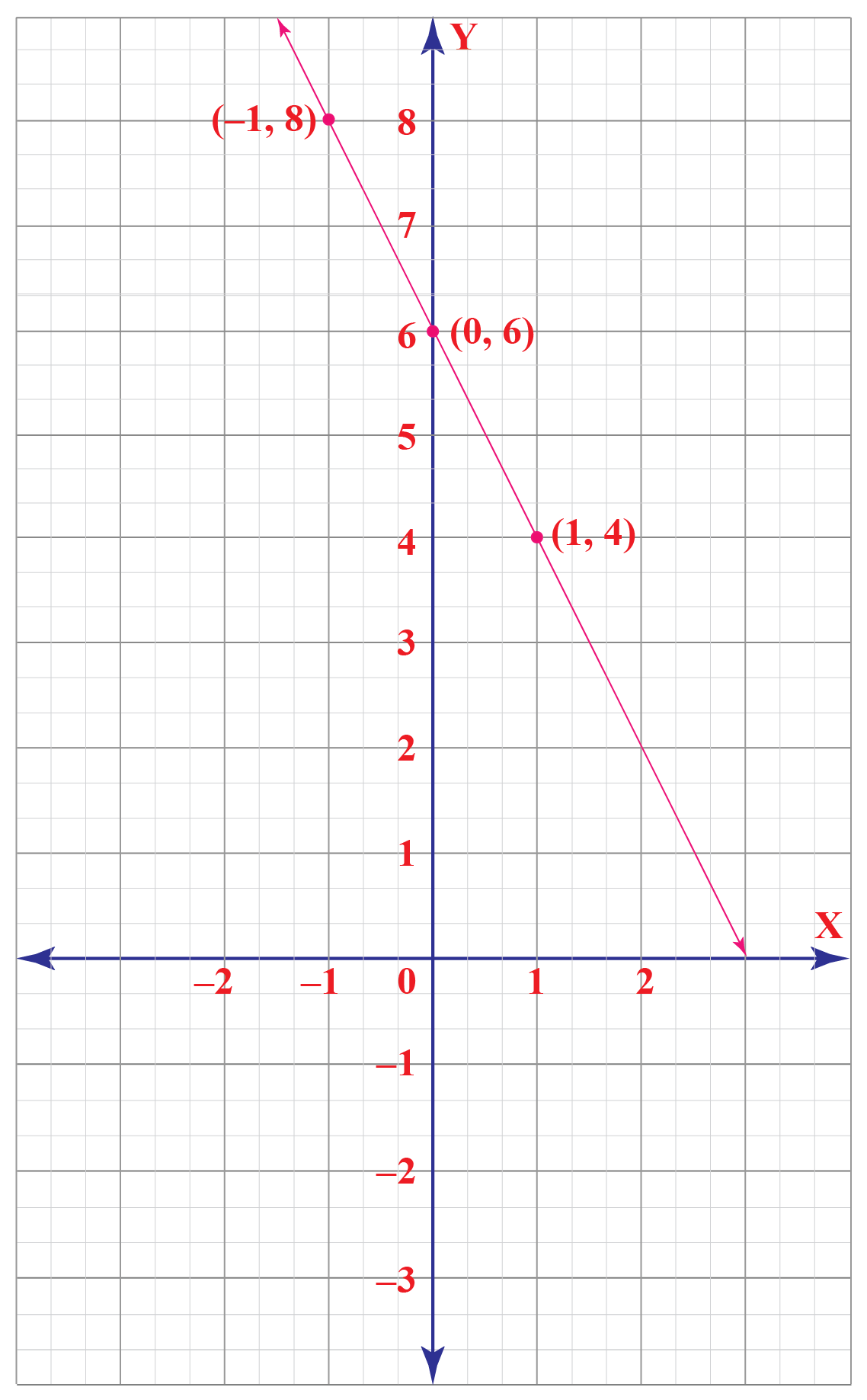### Linear graph calculator

Let's try our hands-on linear graphs. Here is a simulation for two linear equations. You may choose one of them.

Enter the values of ordered pairs (x,y) satisfying the given equation. Press on the "Check" button to verify your answer.

Get ready for some fun while we learn. Let's PLAY along.Tips and Tricks
1. To plot a linear graph, two pairs of (x,y) are sufficient. But, we won't come to know if there is a mistake in obtaining these values as two points can always be joined and they represent a line.  It is advised to plot one more point to ensure that the solutions obtained for the given linear equation are correct.
2. The equation y = kx (where k is a real number) represents a line parallel to X-axis i.e. horizontal line.
3. The equation x = ky (where k is a real number) represents a line parallel to Y-axis i.e. vertical line.

## Solved Examples On Linear Graph

 Example 1

Mrs. Mariot asks John to tell about the nature of the graph of $$3x-7y=16$$ without plotting its values. Can you help John who is struggling to answer this?

Solution

The equation $$3x-7y=16$$ is a linear equation in two variables. That's the 1st thing John needs to observe.

Next, he needs to recall that any linear equation in two variables always represents a line.

These two pieces of information are sufficient to tell about the nature of the graph.

 $$\therefore$$ The given equation represents a linear graph.
 Example 2

Mike has to prepare a linear graph for the equation $$2x+y=8$$ Complete the table given below for this equation.

 x --- 4 -2 y 8 --- ---

Solution

Case 1: $$y = 8 , x = ?$$

\begin{align}2x+y&=8 \\2x+8&=8\\2x&=8-8=0\\x&=0\end{align}

Case 2: $$x = 4 , y = ?$$

\begin{align}2x+y&=8 \\2\times 4+y&=8\\8+y&=8\\y&=0\end{align}

Case 3: $$x = -2 , y = ?$$

\begin{align}2x+y&=8 \\2\times (-2)+y&=8\\-4+y&=8\\y&=8+4\\&=12\end{align}

The solutions are :

 x 0 4 -2 y 8 0 12
 Example 3

Susan is required to draw the graph of $$\,-x+y= 1$$ and check if it's linear. Obtain the graph to check this.

Solution

The equation $$\,-x+y= 1$$ can be rewritten as

$$y = x+1$$

Let's obtain the values of x and y satisfying the above equation.

 x -1 2 1 y 0 3 2

Now, plot these values on a graph.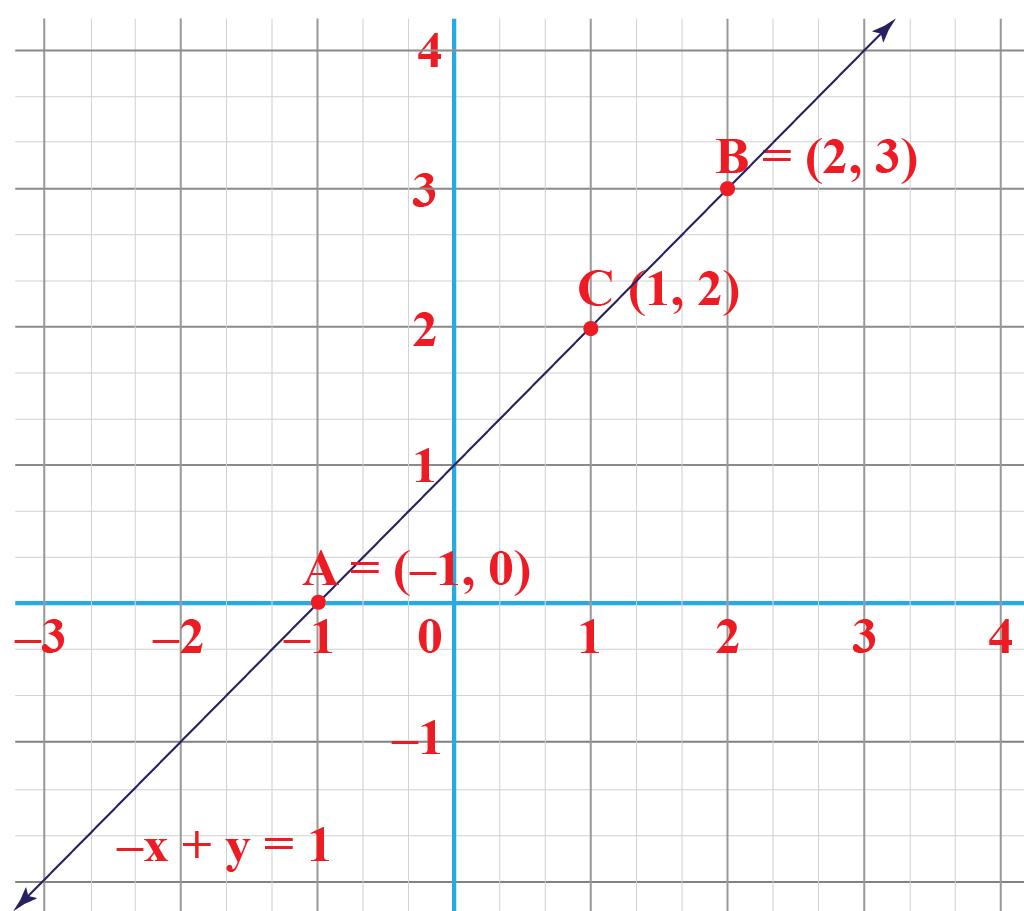$$\therefore$$ The graph is linear.

## Interactive Questions

Here are a few activities for you to practice.

Select/Type your answer and click the "Check Answer" button to see the result.

## Let's Summarize

We hope you enjoyed learning about the Linear Graph with the simulations and practice questions. Now you will be able to easily solve problems on linear graph examples, linear graph meaning, linear graph equation, and linear graph calculator.

## About Cuemath

At Cuemath, our team of math experts is dedicated to making learning fun for our favorite readers, the students!

Through an interactive and engaging learning-teaching-learning approach, the teachers explore all angles of a topic.

Be it worksheets, online classes, doubt sessions, or any other form of relation, it’s the logical thinking and smart learning approach that we, at Cuemath, believe in.

## 1. What is the difference between the line and the linear graph?

Even though both line graphs and linear graphs are made up of line segments, there is a major difference between them.

The difference lies in the figure obtained after joining the line segments. All the points in a line graph are collinear and hence lie on a line.

But in the case of a line graph, they may or may not be collinear.

## 2. Why is a linear graph a straight line?

Consider a linear equation, $$y = mx +b$$.

When we draw a linear graph, its slope(i.e the value of m) is always a constant number. A constant slope denotes a straight line.

Hence, a linear graph is a straight line.

## 3. What are linear graphs used for?

Linear graphs help to understand the trend and predict future values. They are used for various purposes like to check the rate of change of income, production, temperature, etc. over a given time period.

More Important Topics
Numbers
Algebra
Geometry
Measurement
Money
Data
Trigonometry
Calculus